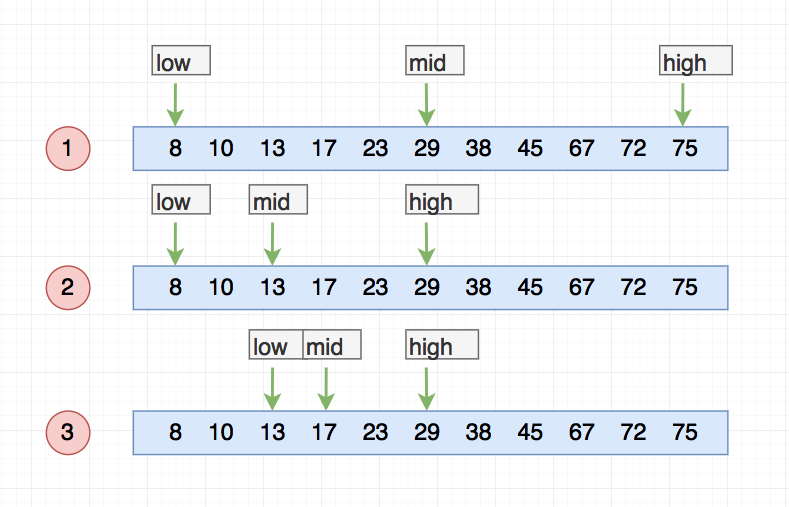# 醉丶春风的Blog

## php二分查找法的基本使用

### 思路

``````\$arr = [8, 10, 13, 17, 23, 29, 38, 45, 67, 72, 75];
``````### 代码实现

#### 非递归查找法

``````/**
* 基础的二分查找
* @param array \$data 一个顺序结构的数组
* @param int \$number 要查找的数据
* @return int 返回元素所在的下标或-1
*/
function binarySearch(array \$data, int \$number) {
// 非正确的参数
if (!is_array(\$data) || empty(\$data)) {
return -1;
}
\$low = 0;
\$high = count(\$data) - 1;

while (\$low <= \$high) {
\$mid = intval((\$high + \$low) / 2);
// mid元素大于给定的元素, 说明可以直接排除从mid开始到high中的所有元素, 将high等于mid-1
if (\$data[\$mid] > \$number) {
\$high = \$mid - 1;
// mid元素小于给定的元素, 说明可以直接排除从low开始到mid中的所有元素, 将low等于mid+1
} elseif (\$data[\$mid] < \$number) {
\$low = \$mid + 1;
} else { // 如果等于mid, 则返回mid
return \$mid;
}
}

return -1;
}
``````

#### 递归查找法

``````/**
* 递归二分查找
* @param array \$data 要查找的元素数组, 必须为有序数组
* @param int \$low 区间起点下标
* @param int \$high 区间终点下标
* @param int \$number 要查找的元素
* @return int
*/
function binarySearchRecursion(array \$data, int \$low, int \$high, int \$number) {
if (!is_array(\$data) || empty(\$data)) {
return -1;
}
// 当low > \$high时, 退出递归
if (\$low > \$high) {
return -1;
}
\$mid = intval(\$low + \$high);
if (\$data[\$mid] > \$number) {
return binarySearchRecursion(\$data, \$low, \$mid-1, \$number);
} elseif (\$data[\$mid] < \$number) {
return binarySearchRecursion(\$data, \$mid+1, \$high, \$number);
} else {
return \$mid;
}
}
``````

#### 需要注意的几点

##### 3.low和high的更新

low=mid+1，high=mid-1。注意这里的 +1 和 -1，如果直接写成 low=mid 或者 high=mid，就可能会发生死循环。比如，当 high=3，low=3 时，如果 a 不等于 value，就会导致一直循环不退出。

#### 优化后的代码

``````/**
* 优化二分查找
* @param array \$data 一个顺序结构的数组
* @param int \$number 要查找的数据
* @return int 返回元素所在的下标或-1
*/
function binarySearch(array \$data, int \$number) {
// 非正确的参数
if (!is_array(\$data) || empty(\$data)) {
return -1;
}

\$low = 0;
\$high = count(\$data) - 1;

// 给定元素不在给定的区间范围内, 直接返回
if (\$number < \$data[\$low] || \$number > \$data[\$high]) {
return -1;
}

while (\$low <= \$high) {
// 更新计算mid元素值
\$mid = \$low+((\$high-\$low)>>1);
// mid元素大于给定的元素, 说明可以直接排除从mid开始到high中的所有元素, 将high等于mid-1
if (\$data[\$mid] > \$number) {
\$high = \$mid - 1;
// mid元素小于给定的元素, 说明可以直接排除从low开始到mid中的所有元素, 将low等于mid+1
} elseif (\$data[\$mid] < \$number) {
\$low = \$mid + 1;
} else { // 如果等于mid, 则返回mid
return \$mid;
}
}

return -1;
}
``````

## 最新回复